﻿ Spin-orbital interactions in spintronics are wrong.

# Spin ? Spintronics ? Fantasy ?

## Why do top journals and researchers believe "fantasied" spin even now ?

### [ Point-like electron CANNOT spin ! ]

(Fig.1) Spinning speed of an electron is much faster than light !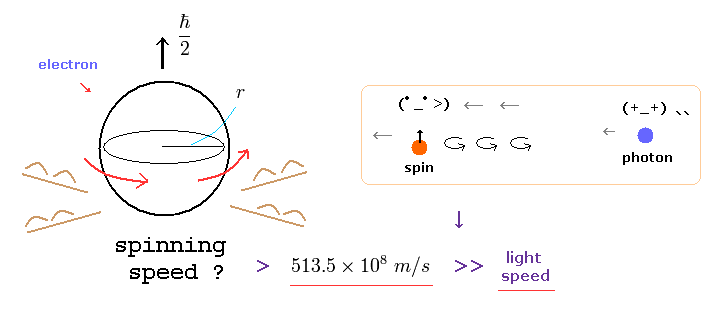According to actual experiments, a single electrons is a very small point-like particle.
But quantum mechanics insists this point-like electron has 1/2 ħ angular momentum.
As you know, the angular momentum means mv× r ( m= electron mass, v=rotation speed, r is electron radius ).

Considering electron's very small mass and radius, this rotating speed "v" must be more than 100 times light speed to reach 1/2 ħ ! ( See also this page. )
This means quantum mechanical spin is unrealistic and just a fantasy.

### [ Reason why strange spin is fantasy and wrong. -- part II. ]

(Fig.2) Fermions CANNOT return by one 360-degree rotation !Strange to say, according to unrealistic quantum mechanics, all fermions such as electron, neutron, and proton CANNOT return to their original states by 360-degree rotation !
Because these particles has spin angular momentum 1/2ħ.
Of course, if this acgular momentum is an integer times ħ like Bohr's orbit, it can return by 360-degree rotation.
( This is a quite natural thing. )

Surprisingly, as shown in this page, this fantasied phenomenon was actually observed in experiments, they insist.
The important point is that these experiments assume that fermions have 1/2 ħ as precondition.
If we change this one-side assumption, and suppose they have natural ħ angular momentum. these experiments only showed particles return by 360-degree rotation.
So these experiments clearly showed spin angular momentum 1/2 ħ is wrong and fantasy.

### [ Why electron spin magnetic moment is "Bohr magneton" like Bohr's orbit ? ]

(Fig.3) Bohr's orbit (= Bohr magneton ) = electron spin (= Bohr magneton ) ?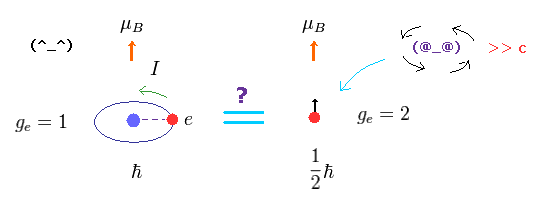As shown in this page, the magnetic monent of Bohr's ground state orbit is Bohr magneton.
Surprinsingly, electron spin magnetic moment is ALSO this Bohr magneton !
( Though spin has NOTHING to do with Bohr's orbit. )

Because the ground state of quantum mechanical hydrogen has NO angular momentum, and instead has this spin.
The experimental value of this hydrogen magnetic moment is just Bohr magneton gotten from Bohr's orbit.
So they started to insist if electron "spin" also has Bohr magneton, it agrees with experimental results.
That's all.

### [ Strange "spin" has the most influential power in the present world ! ]

(Fig.4) If strange spin is wrong, almost all theories become dead.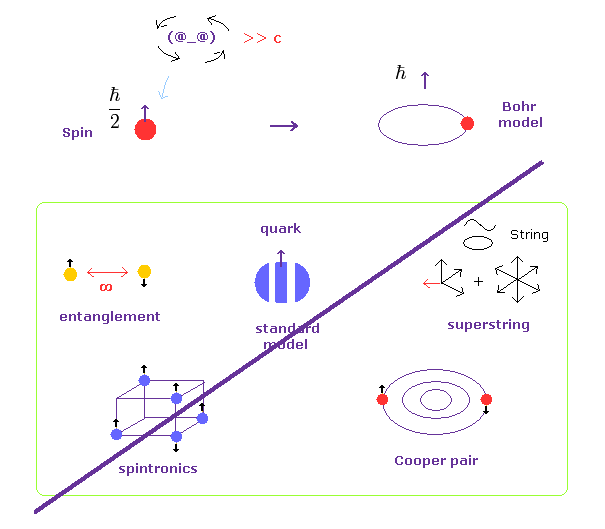Though strange spin is very unrealistic and unreasonable, top journals and researchers NEVER give up this "spin", and NEVER ask what "spin" really is.

Because if this spin is wrong, and changed into usual classical circular orbit, all theories such as entanglement, standard model, superstring, spintronics, and Cooper pair would collapse.
So in fact, this methematical "spin" has the greatest influence in fictions existing in the present world !

Of course, considering these theories are all unrealistic and problematic, we have to revise all these concepts, and proceed to develop "real" science.
It's very easy. Just stop "Shut up and calculate !" about what spin really is.

## Reason why "spin" in condensed matter physics is wrong.

### [ Spin-spin interaction is too weak to cause ferromagnetism. ]

(Fig.5) Spin - spin interaction is illusion !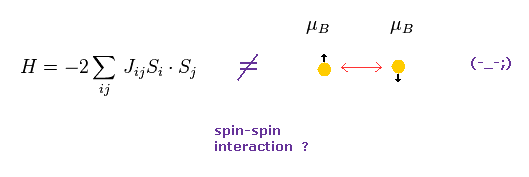In the present condensed matter physics, they often use the relation fo Fig.5 to describe ferromagnetism and diamagnetism.
In Fig.5, when the exchange interaction constant J is positive, parallel spins make energy negative and stable (= ferromagnetism ).

So they insist one spin influences other spins in ferromagnetism.
But if spin means "magnetic moment" (= Bohr magneton ), this ferromagnetism is impossible.

(Fig.6) Spin - spin interaction is unrealistically weak.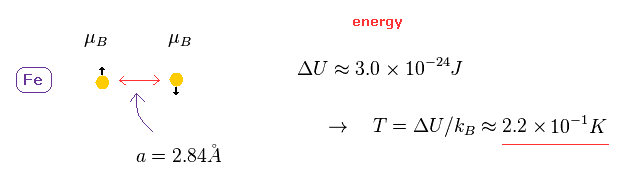The lattice constant of iron is 2.84 Å.
In this distance, the energy difference between parallel and antiparallel spin interactions are too small, as shown in Fig.6.
Using Boltzmann constant k, this energy difference is equal to only 0.22 kelvin. ( See appendix. )

So if spin-spn interaction is caused by their magnetic moments, a magnet is easily broken in room temperature !
According to this book ( Story of Spin by S.tomonaga ), the energy difference between singlet and triplet spectrums cannot be explained only by spin-spin interaction.

So they just change the name of some Coulomb energy difference into spin-spin interaction !
( Officially, these energy differences are caused by "antisymmetry" or "symmetry" of wavefunctions. )

### [ Spin-orbit interaction in spintronics is an illusion ! ]

(Fig.7) Real spin state ? Point particle ?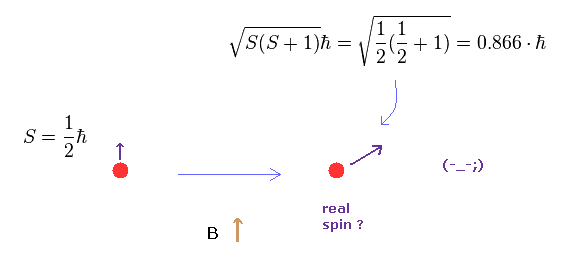In spintronics and condensed matter physics, they often use the relation of Fig.7.
So according to quantum mechanics, "real" spin angular momentum is NOT 1/2 ħ !
Strange to say, they insist point-like spin is precessing, and almost leans toward the direction different from magnetic field B.

This spin relation of Fig.7 is NOT gotten from Schrodinger's hydrogen, different from usual anglar momentum L.
And they cannot explain why this angle of inclination is always constant even in different magnetic fields.
The origin of this mysterious inclination remains a riddle even in 21th century now.
This is strange.

### [ Lande g-factor doesn't agree with experimental results. ]

(Fig.8) Proof of spin ?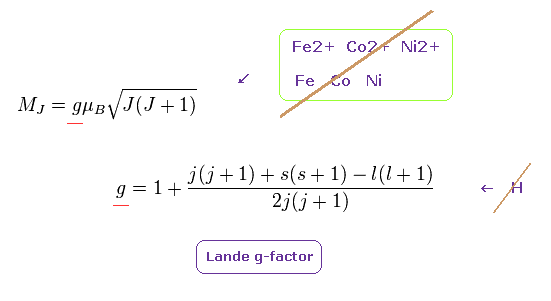As shown in this page, complicated Lande g-factor is gotten mainly from anomalous Zeeman effect of sodium.
First, this Lande g-factor does NOT apply to simple hydrogen atom, because when Lande introduced this relation, they believe S means total magnetic moment of inner core electrons ( NOT spin ! ).

Furthermore, this Lande g-factor doesn't agree with experimental results in important metals and ions such as Fe, Co, Ni, Fe2+, Co2+, Ni2+, Sm...

### [ They "artificially" delete original spin-orbital interactions. ]

(Fig.9) Angular momentum is zero ?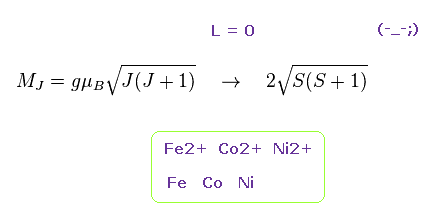To fit Lande g-factor to experimental results, they artificially erase angular momentum ( L=0 ) in 3d electrons in Fe2+, Co2+, Ni2+.
They insist 3d electrons of these ions are freely flying around, NOT bound to nuclei, so their angular momentum become zero.

But this interpretation is strange, because 3d orbitals have large angular momentum, and they are attracted towards 2+ positive ions ( If we remove 3d electrons, Fe2+ becomes Fe4+, Fe5+ .. Impossible ! )
These manipulations are clearly only to make the calculation results close to experimental results.

Furthermore, atoms such as Fe, Co, Ni, Pm, Sm .. are completely inconsistent with this Lande g-factor even after these manipulations.
So they started to introduce various other effects caused by other atoms. ( ex. other "artifical" spin-orbit interactions. )
The present spintronics is completely dependent on these various artificial manipulations.

### [ 3d, 4s, 4f, 5d, 6s orbitals are real ? ]

(Fig.10) We CANNOT directly see these orbitals !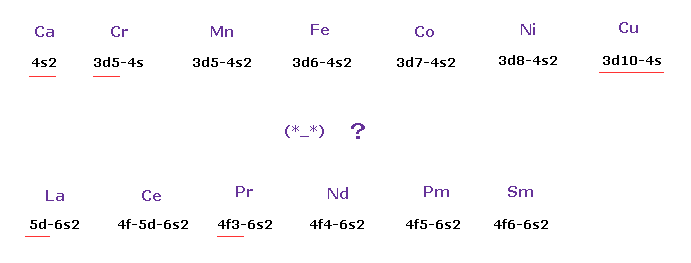As shown in Fig.8 this Lande g-factor is completely dependent on combinations of various orbitals ( s, p, d, s ... )
The problem is whether these orbitals and electron's arrangements are really correct or not.
For example, in K and Ca atoms, electrons first enter 4s orbitals.
And in other atoms after that, electrons can enter 3d orbitals. True mechanism remains a riddle.

In Lanthanoids, orbitals are 5d-6s2 in La, 4f-5d-6s2 in Ce, 4f3-6s2 in Pr ..... ??
These orders are very complicated !
The important point is that we cannot see these orbitals in actual experiments.
Because, in coordinate bonds, these fictional orbitals become mixed into other things like sp2, sp3..
They can artificially define these orbitals to agree with various spectrum results.

### [ Angular momentum zero = 4s, 5s, 6s ... are impossible in actual atoms. ]

(Fig.11) Angular momentum zero (= s state ) → outer electron penetrates ??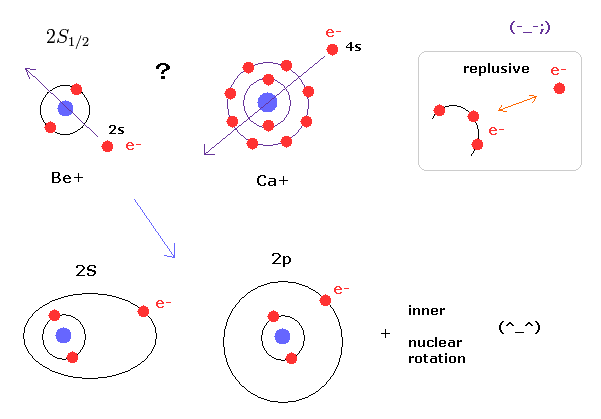As I said many times, the "s" state with zero angular momentum ( L=0 ) is impossible in real atomic structures.
Because outer electron cannot penetrate inner electrons due to the strong repulsive Coulomb forces.

Instead of admitting this strange zero angular momentum, it is more natural that we think the outer electron is orbitting around the inner electrons. ( NOT penetrating them ! )
If we consider elliptic and circular orbits, we can explain the energy difference between 2s and 2p states, and on the other hand, Dirac's hydrogen includes wrong orbitals, as shown in this page.

### [ "3d" orbitals really exist ?? ]

(Fig.12) 3d orbitals are very doubtful.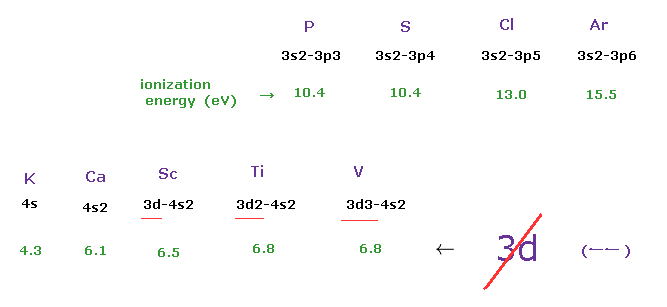As shown in Fig.12, the first ionization energies become slightly bigger as P → S → Cl → Ar.
And in Potassium (K), this ionization energy becomes suddenly smaller (= 4.3 eV ), which means different orbital starts from K.

Ionization energies are smoothly increased in 4s and 3d mixed orbitals. ( 4.3 → 6.1 → 6.5 → 6.8 .. )
Considering these ionization energies, it is natural that we think 4s and 3d are in the same "n" energy number.
So this definition of "3d" is very unnatural.

Actually, to get magnetic moments of V4+, V3+, V2+, Cr3+ ..., they artificially make angular momentum zero (= ignoring Lange g-factor ), though they include 3d orbitals.
These magnetic moments are about 2 (= V4+), 3 (= V3+ ), 4 (= Cr3+ ), 5 (= Cr2+ ) times Bohr magneton.

### [ Ferromagnetism and diamagnetism ]

(Fig.13) What causes ferromagnetism ?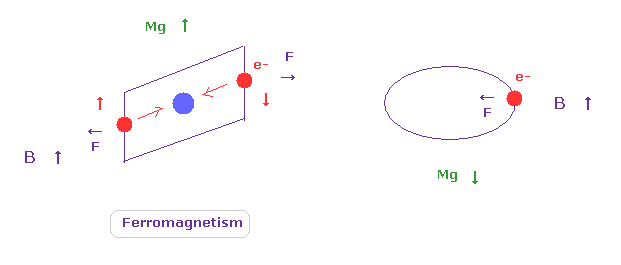Even in unrealistic quantum mechanics, classical Lorentz force is very important.
As shown in Fig.13 left, when outer electrons are loosely bound to nuclei, their magnetic moments turn in the same direction as the external magnetic field (= B ).

The important point is that these materials showing ferromagnetism need to be regularly arranged crystals.
In most materials and molecules, magnetic moment of each electron ( orbit ) turns in various direction, which cancels eath other and causes diamagnetism.

Of course, due to crystals, de Broglie waves are thought to synchronize with each other, too.
Because, as shown in helium in top page, it is natural to think that magnetic field is caused by de Broglie waves (= electron's motion ).
In superconductivities, due to completely free electrons and Lorentz force, they show strong diamagnetism, as shown in Fig.13 right.

## BCS theory and Cooper pair are wrong.

### [ They should clarify vague "phonons" as soon as possible. ]

(Eq.1) What are "Phonons" ? → Shut up and calculate !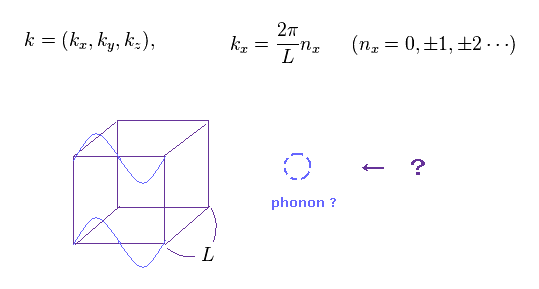In condensed matter physics, they often use very vague concepts of phonons.
Consider a cube of side L, and wavelength satisfying boundary condition, they suppose the relation of Eq.1.

(Eq.2) Phonons mean de Broglie waves !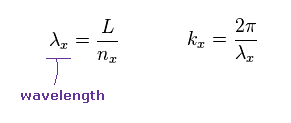Here λ is wavelength of phonon, and k means wave number.
These relations indicate these vague phonons are equal to some real de Broglie waves.
So in condensed matter physics, they tacitly depend on some de Broglie waves.

(Eq.3) Number of resonating modes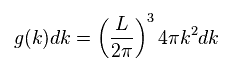Here we get the total number of oscillating modes included in spherical shell of wave number k, as shown in Eq.3.
This relation is often used in lattice models of condensed matter physics.

### [ Superconductivity and London equations are classical Maxwell theory. ]

According to Meissner effect, the magnetic field inside superconductivity becomes zero.
These effects could be explained by usual classical Maxwell equations by London.

(Eq.4)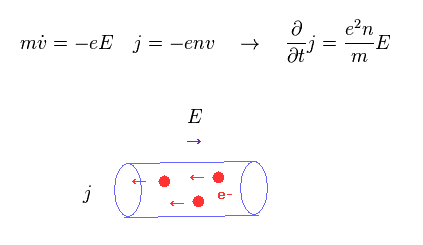In Eq.4, electron density is "n", and electron's velocity is "v".
In the electric field E, moving electrons (= current density "j" ) are accelerated.

(Eq.5) London equation = diamagnetism.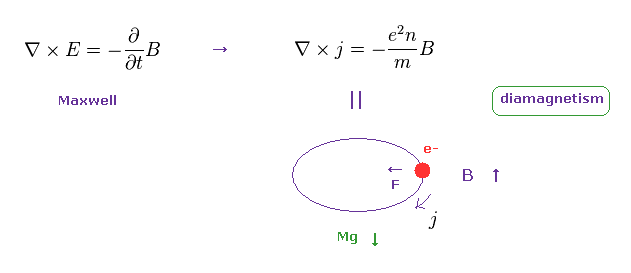Using Eq.4 and Eq.5 (= Faraday's law ), we get London equation of Eq.5, which shows (perfect) diamagnetism of superconductivity.
Because according to Faraday's law, moving charge tries to cancel external magnetic field obeying classical Lorentz force.

(Eq.6) Other Maxwell equations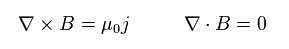Here we use other Maxwell equations of Eq.6.
Combining all equations above, we get

(Eq.7)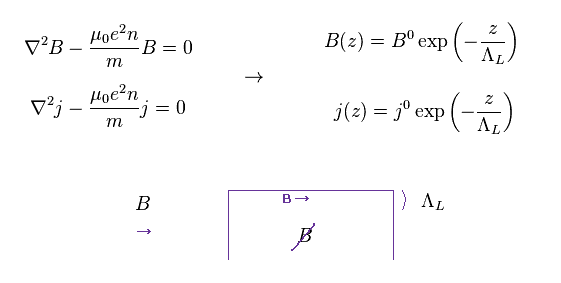where
(Eq.8)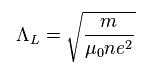ΛL is London penetration depth, which means how deeply external magnetic field can enter superconductivity.
So external magnetic fields are more decreased inside the superconductivity.

As shown in Faraday's law and Maxwell equaions, this Meissner effect is completely caused by classical electromagnetism

### [ Repulsive two electrons attract each other ?? → Cooper pair ? Phonon ? ]

(Fig.14) "Cooper pair" really exists ??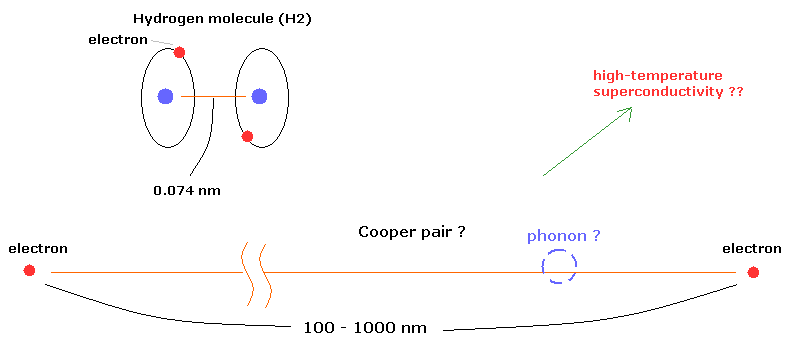According to the present condensed matter physics, it is said that a Cooper pair is two electrons attracting each other at low temperature through very vague existence of "phonons".
Surprisingly, the distance between the two electrons in the Cooper pairs is about 100- 1000 nanometer (nm) !
For example, in the hydrogen molecule (H2), the distance between two nuclei is about 0.073 nm.
How can these two electrons bind stably to each other in the superconductor, overcoming repulsive Coulomb force ? This is strange.

The important point is that phonons they introduced are very artificial concepts.
And these phonons are only "mathematical" products ( Not real onject ), so the present BCS theory cannot be more developed escaping from this mathematical restrictions. ( See also this page. )

(Eq.9)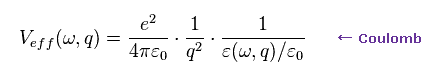Here we simply explain why these phonons are very artificial and only mathematical ( NOT physical ) concepts.
Eq.9 is Fourier components of replusive Coulomb force between two electrons.
They intentionally change permittivity (= ε ) to add attractions by phonons, as follows,

(Eq.10)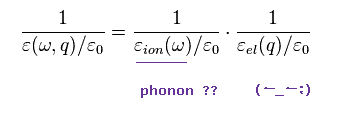where they defined the following relations by force,

(Eq.11)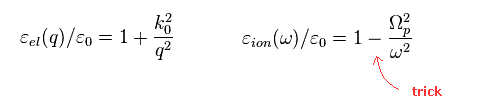In Eq.11, the negative sign is the point of this attraction by phonons.

Strange to say, in Eq.11, Ωp means phonon's angular frequency, they insist.
But is it allowable that they suddenly introduced this vague concept to explain attractive force ?

(Eq.12)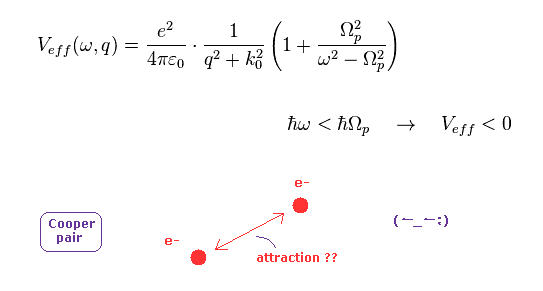Substituting Eq.10 and Eq.11 into Eq.9, we obtain Eq.12.
In Eq.12, when electron's energy ( ħω ) is less than fictional phonon's energy (= ħΩ ), this repulsive Coulomb force is changed into attraction !

But as you feel, this attraction between two electrons (= Cooper pair ) was suddenly introduced without offering reasons.
So these Cooper pair and phonons are only speculation, and does NOT prove the existence of strange "spin" at all.

### [ Quantized magnetic flux really means Cooper pair ?? ]

Here we show quantized magnetic flux is caused by real de Broglie waves, and it doesn't mean Cooper pair.
Because phonons themselves are unreal quasiparticles, and the attractions between two electrons are very unnatural.

(Fig.15)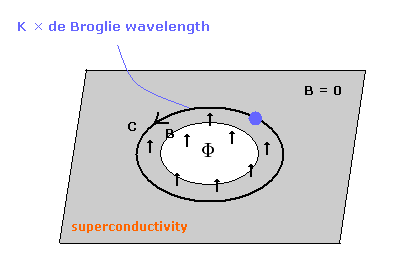The magnetic flux quantum (Φ0) is the quantized magnetic flux passing through the hole of a superconductor in Fig.15.
This maxgatic flux is known to become an integer times the follwoing quantized value.
( So, Φ = Φ0 × an integer. )

(Eq.13)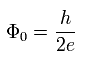First we try to get Eq.13 using Schrodinger equation.
To get Eq.13 in Schrodinger equation, we must hypothesize the "Cooper pair", which consists of two electrons.

In the superconductor at very low temperature, phases of the all electrons become almost same, as shown in Eq.14.
Unfortunatelly, they try NOT to clarify what this phase of electron really is.
(= Again, Shut up and calculate ! )

(Eq.14)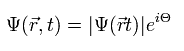where the phase (Θ) consists of momentum (p) and energy (E), as follows,

(Eq.15)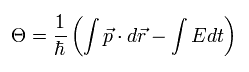If we forget the repulsive force between two electrons, and suppose the "Cooper pair (= -2e )", the momentum (p) and the energy (E) become
(Eq.16)where considering the Lorentz force, we use magnetic potential (= A ), and electric potential (= φ ).

When we pay attention to only the space part, Eq.15 is
(Eq.17)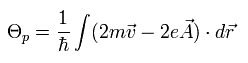If we consider the phase of the wavefunction returns to its original, by rotating it around the hole of Fig.15,
(Eq.18)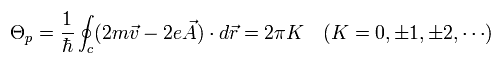wehre K is an integer.

According to the Meissner effect, the magnetic field and electron's current in an superconductor can be ignored.
So we can suppose the electron's velocity (= v ) of Eq.18 is zero, as follows,
(Eq.19)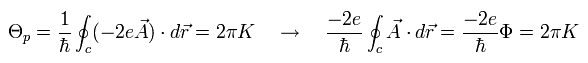where Φ means the total magnetic flux surrounded by the vector potential (= A ) according to Maxwell's law.
( B = ∇ × A.   Φ = BS. )

From Eq.19, the magnetic flux (Φ) in the hole becomes quantized, as follows,

(Eq.20)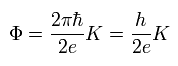"K" is an integer.
Here we can define quantized magnetic flux unit, as follows,

(Eq.21)Eq.21 is called "magnetic flux quantum (Wb)".
So they insist Cooper pair can explain quantized magnetic flux.
But they don't explain what the wave phases of Eq.14 really is.
( Of course, as you notice, these phases are related to real de Broglie waves. )

### [ Real de Broglie wave can explain quantized magnetic flux naturally. ]

(Fig.16) An integer times de Broglie wavelength.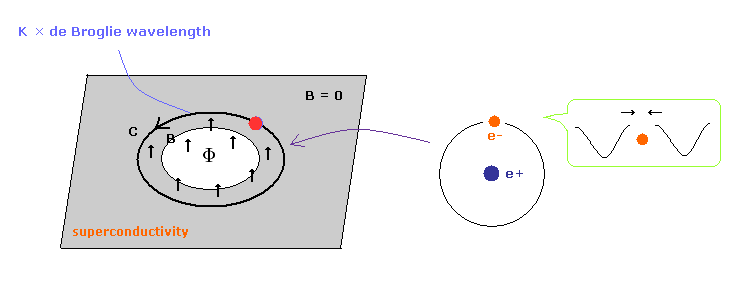Here we suppose the electron is rotating around near the hole of Fig.16 to cancel the magnetic field.
When the centrifugal force is equal to the Lorentz force,
(Eq.22)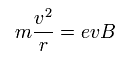When one round of the orbit is equal to an integer (= K ) times de Broglie's wavelength,
(Eq.23)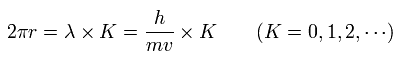where λ = h/mv.

From Eq.22 and Eq.23, we have
(Eq.24)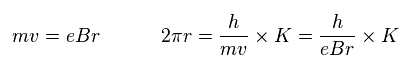So,
(Eq.25)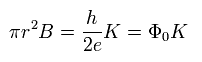Eq.25 means the magnetic flux (Φ) passing through the hole of Fig.16
( πr2 is the area of the hole. )
As a result, we can get the magnetic flux quantum of superconductor using "real" de Broglie waves.

(Eq.26) = Eq.21.And Cooper pair is not needed here.
Of course, if Cooper pair does not exist, the problem of high-temperature superconductivity never happen from the beginning.
( They try to solve this problem using conformal field theory of the string. But these methods have NO reality. )

This de Broglie wave's phase is related to quantum tunnel and Josephson effect.
So quantum tunnel is caused just by real de Broglie waves ( NOT fantasied quantum mechanics ).

## Real De Broglie waves are important in condensed matter physics.

### [ Fractional quantum hall effects can be explained by de Broglie waves. ]

(Fig.17)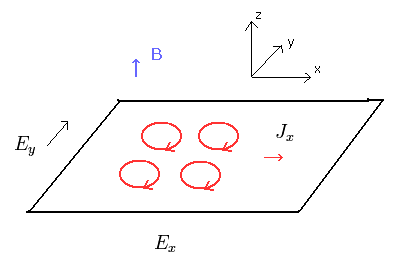In quantum hall effects, electrons are moving in the dirrection perpendicular to external electric and magnetic fields.
As shown in this page, we can explain these phenomena including fractional quantum hall effects by real de Broglie wave's quantization.

(Fig.18) Conductivity ( currents ) are quantized.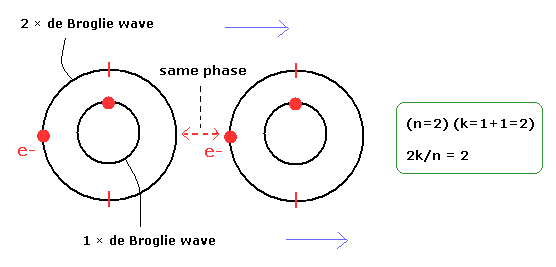In quantum hall effect, conductivity ( and current ) is quantized.
Considering electron's de Broglie waves, it is obvious that their wave phases are related to these effects.

### [ "Anyons" with fractional charge = quarks ?? ]

(Fig.19) Why strange fractional charges appear ONLY in 2D system ??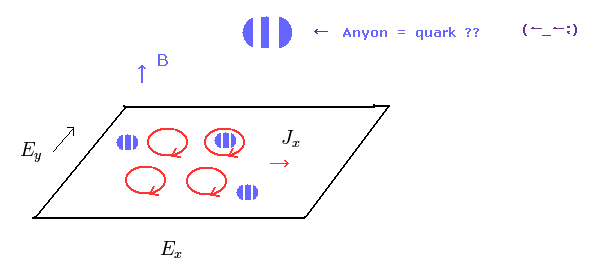The true mechanism of fractional quantum hall effect remains unknown.
In some theories, they introduced very strange particles called "anyon".

Anyons are neither bosons nor fermions !
Furthermore, these anyons have fractional charge ! Impossible.
As you notice, the present quantum mechanics tends to be just a fantasy ( from the beginning ).

### [ Spin hall effect is caused by orbital motions NOT by imaginary "spin". ]

(Fig.20) Spin hall effect ? Really "spin" ?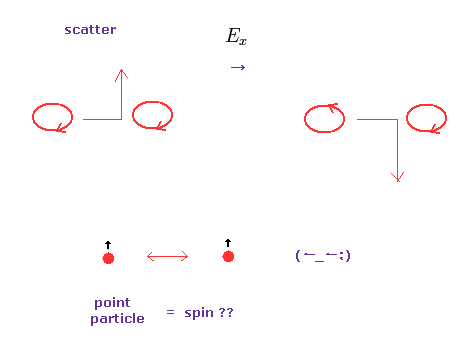Spin hall effect is completely different from usual hall effect.
Moving electrons turn in the direction perpendicular to electric field ( no magnetic firld ), dependeng on their magnetic moment's direction ( up or down ).
Some "scattering" between particles are thought to be related to this effect.

This effects is much weaker than usual hall effect, so very difficult to detect.
They insist these magnetic momemts are caused by "spin".
But how they distinguish directions of them, though electrons are point-like particles ? Impossible.
First, very tiny point-like particles cannot collide with each other.

It is natural that we think the difference of directions in orbital motions (= de Broglie waves ) causes this effect.

## Spin-orbit coupling is illusion.

### [ Spin - magnetic interaction of quantum mechanics is only mathematical "trick". ]

(Fig.21) Spin magnetic interaction is an illusion !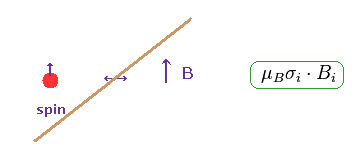In quantum mechanics, spin orbit interaction ( coupling ) is derived from Dirac equation and electromagnetic interaction.
In fact, in the process of this calculation, they use a kind of "mathematical" trick.
In this calculation, they artificially change de Broglie wavelength of an electron into external magnetic field !

As you feel, de Broglie wavelength of an electron has NOTHING to do with external ( not internal ) magnetic field B.

(Fig.22) Lorentz force → replacements.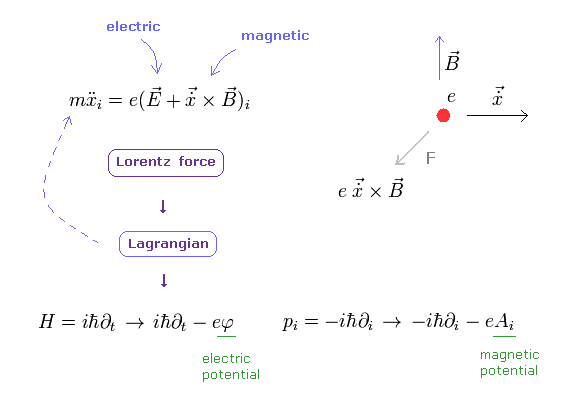The electromagnetic interactions in quantum mechanics and electrodynamics (QED) completely depend on classical Lorentz force.

As shown in this page, first they get Lagrangian which gives Lorentz force.
Second, they derive Hamiltonian (= H ) based on this Lagrangian.
In this final Hamiltonian, magnetic (= A ) and electric (= φ ) potentials are added to the original equations of motion.

(Fig.23) Free-particle equation → A, φ potentials are added → spin-magnetic interaction ??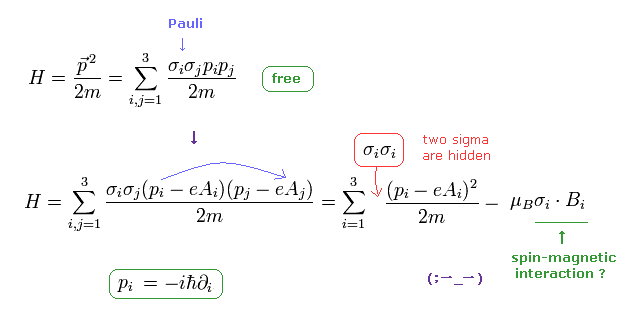The first equation of Fig.23 is Hamiltonian of free particle.
σ is Pauli matrices (= spin ), which is hidden in the first equaion.
The second equation is gotten using replacements of Fig.22 last line (= external electromagnetic fields ).

They insist spin magnetic interaction term can be obtained in this calculation. But is it really so ??
( Here, μB is "Bohr magneton". )

(Fig.24) de Broglie wave of an electron is changed into "external" magnetic field ??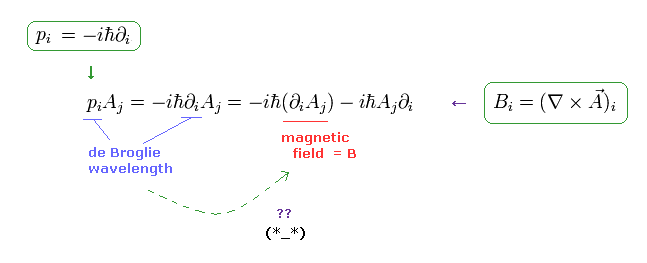In Fig.23, they use the differential operator (= de Broglie wavelength ) of an electron as a part of external magnetic field !
Magnetic field B is equal to ∇ × A (= magnetic potential ).
( This ∇ is originally "de Broglie wave" of an electron. )

Of course, external magnetic field B is completely independent value from this electron.
So this spin-magnetic interaction in quantum mechanics is completely a "mathematical" trick and wrong.

### [ Spin - orbit interaction is "too weak" comparing experimental values. ]

(Fig.25) Spin - orbit interaction is an illusion !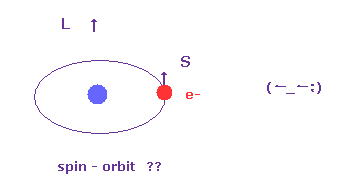Spin-orbit inteaction is also gotten from Dirac equation approximately (= Pauli approximation ).
In fact, the strength of this spin-orbit interaction of Dirac equation is too weak in comparison with experimental values.
( This means spin-orbit interaction is NOT a true thing. )

So they insist some other strong electric potential may be generated by some band structure of metals.
The present condensed matter physics tends to escape out of "real" mechanism into very vague concepts and explanations.
They NEVER try to seek clearer mechanism about these phenomena at all.

(Fig.26) Spin - orbit interaction is an illusion !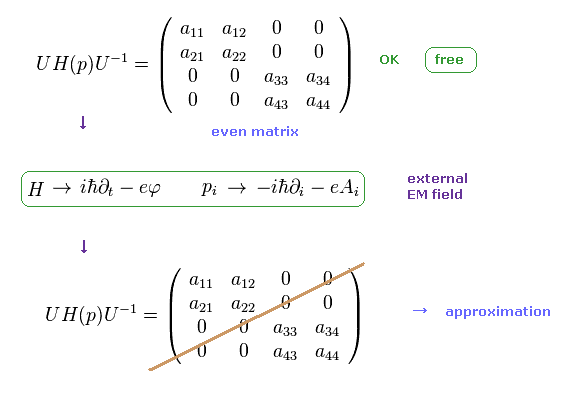As shown in this page, Dirac spinors (= fermions ) are 4 × 1 matrices.
And the upper and lower spinors are mixed, which is completely differenct from usual non relativistic quantum field theory.

So they need to get even matrices from Dirac matrices using diagonal matrix U, as shown in Fig.26 upper.
But when Hamiltonian H includes external magnetic field (= A, φ ), they cannot get even matrices.
So they need to rely on some approximation.

(Fig.27) Approximation → spin - orbit interaction term really appears ?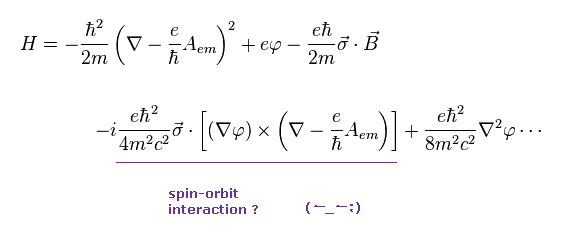Relying on some approximation (= Pauli approximation ), spin-orbit interaction term can be gotten, they insist.
The important point is that this spin-orbit interaction based on relativistic quantum field theory is much weaker than actual experimental values.

(Fig.28) Comparison of spin-orbit interactions.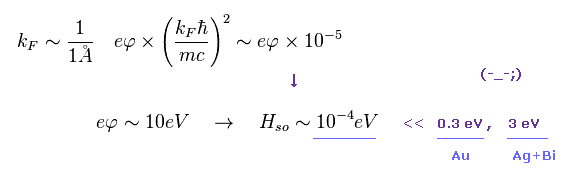In Fig.28, kF is approximate wave number, electric potential (= eφ ) is supposed to be about 10 eV (= outer electron ).
Using these values, spin-orbit interaction by Dirac equation becomes about 10-4 eV, very weak.

This value is much weaker than experimental values in the surface of Au or Ag+Bi ( 0.3 eV and 3 eV, respectively ).
So they artificially change spin-orbit interaction coefficient into much bigger one in various metals.
( It means these are "free" parameters. )
In conclusion, spin-orbit interaction is a "theoretically" and "experimentally" ungrounded concept.

### [ Mysterious Pauli exclusion principle is much stronger than all other 4 fundamental forces ! ]

(Fig.29) Pauli exclusion principle is a force ? → "Shut up and calculate !"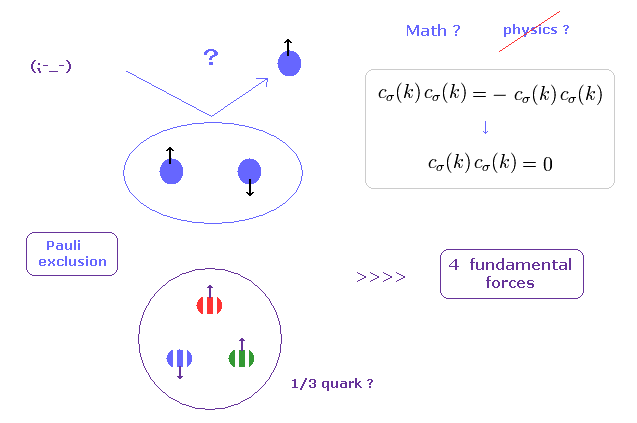If you see some condensed matter physics textbooks, you would find those books are filled with unrealistic "spins".
Main reason is "Pauli exclusion principle", in which two identical fermions cannot enter in the same place (= state ).
( But I think, this definition of the "same" place is very vague. How can we define these boundary lines ?? )

For example, in 1s energy state, only spin "up" and "down" electrons can exist.
They introduced very artificial "colors" (= red, blue, green ) in quarks to satisfy Pauli exclusion principle by force.
So, the force involved in this mysterious Pauli exclusion principle is much stronger than all other fundamental forces !

The problem is physicists NEVER try to ask what this Pauli exclusion principle really is, even in 21th century now.
Instead of wasting a great deal of money in useless accelerator, they should investigate the true form of this Pauli exclusion, first, I think.

## True form of "Spin" and "orbital" is de Broglie wave.

### [ Spin-spin interaction is too weak to cause ferromagnetism. ]

(Fig.30) Spin - spin interaction is illusion !As shown in Fig.5 and Fig.6, we explain spin-spin interaction is too weak to cause ferromagnetism.
So they inisist strong exchange interaction constant J is the result of some vague "quantum" mechanical effects.

So the word of "quantum mechanical" effects is very convenient to express unkown phenomena !
They must clarify these vague concepts including unrealistic "quasiparticle" as soon as possible.

(Fig.31) "Synchronizing" de Broglie wave is true nature of ferromagnetism.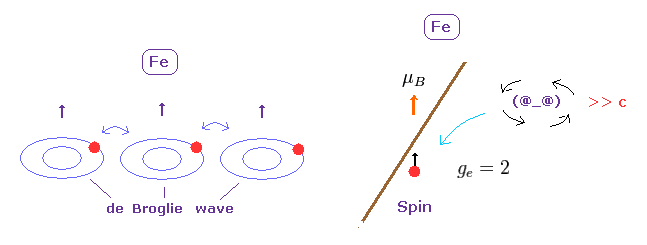If strange "spin" magnetic moment is too weak to cause strong ferromagnetism, there is ONLY one thing left to explain this phenomenon.
As you notice, when de Broglie wave caused by each electron's motion is synchronizing with each other, the stability of ferromagnetism can be naturally explained.

As I said above, the present condensed matter physics tacitly depends on this de Broglie waves as "phases" or wave number k.
( But they NEVER admit this realistic wave. Instead they just do "Shut up and calculate !" ).

(Fig.32) Electron spin is an illusion.Because as shown in this page, the instant they start to ask what this spin really is, they would notice this unrealistic spin is just a classical orbital motion.

And it's too late for physicists to correct this spin, because denying "spin" means rejecting all quantum mechanics and quantum field theory.
As a result, they are wandering around imaginary extradimensions and another worlds "forever" to explain "quantum" world.

### [ 3d-4s spin-spin interaction is NOT real. ]

(Fig.33) s-d interaction is too weak.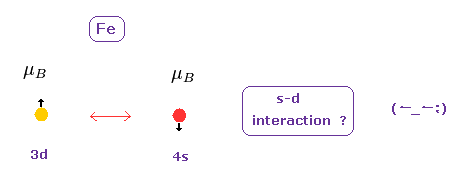It is said that "spins" of 3d and 4s electrons are interacting with each other and cause various macro effects in materials.
But like Fig.30, this s-d spin interaction is too weak, when we think spin magnetic moment is involved in this effect.

(Fig.34) s-d interaction is too weak.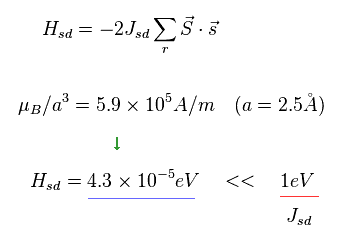Lattice interval is supposed to be 2.5 Å.
In this interval, when they try to get some energy value using "spin" magnetic moment, this energy becomes too weak in comparison with actual interaction (= 1 eV ).
So again they insist this is due to some other "quantum mechanical" phenomena.

But as you feel, strange "spin" itself is just an imaginary concept from the beginning.
This fact clearly shows that these strong s-d interaction are NOT by "spin", but by some other things such as de Broglie waves.

### [ Anisotropic magnetoresistance (AMR) is NOT "illusory" spin-orbit interaction. ]

(Fig.35) AMR is relatied to different de Broglie wave's directions.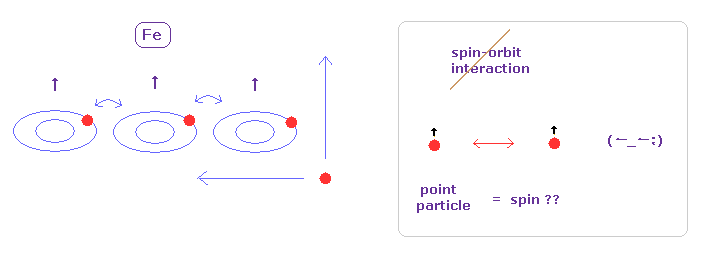In ferromagnetic materials, it is known that electrical resistance changes depending on the relation between magnetic and current's directions.
This phenomenon is called "anisotropic magnetoresistance" (= AMR ).
They insist the ferromagnetism is caused by spin of "3d" electrons of metals such as Fe.
So they insist this AMR is the result of spin and orbit (= current ) interactions

But as shown in Fig.6 and Fig.28, it is obvious that some other things different from "spin" is playing a main role in these ferromagnetism and spin-orbit interactions.
So it is quite natural that we think AMR is caused by de Broglie wave's direction, NOT by point particle's spin.

### [ Giant magnetoresistance (GMR) is also caused by de Broglie waves. ]

(Fig.36) GMR is spin-orbit interaction or scattering ?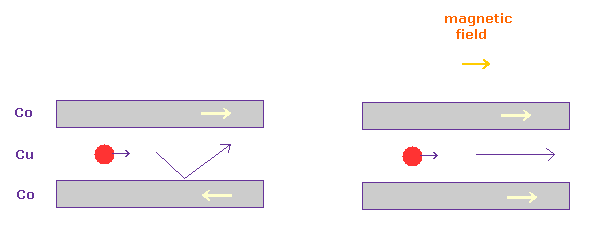In Fig.36, very thin (= 10 Å) nonmagnetic layer (= Cu ) is sandwiched by some magnetic material (= Co, Fe ).
In this case, when magnetic directions in upper and lower Co layers are different from each other (= Fig.36 left ), the electric resistance in Cu layer becomes too strong.
This phenomenon is called "giant magnetoresistance (= GMR )".

It is said this GMR is caused by some electron's scattering or spin-orbit interactions.
But this mechanism remains unknown.
Considering the fact that ferromagnetism happens by some other things different from "spin", it is natural that we think GMR is also caused by de Broglie wave's directions.

### [ Hall effect is classical electromagnetism. ]

(Fig.37) Hall effect.In hall effect, charged particle moves in the direction, which is perpendicular both to magnetic (= B ) and electric (= E ) fields.
This phenomenon is explained by usual classical Lorentz force ( see some hall effect textbooks ).

In Fig.37, electric force ( eE ) is just equal to magnetic Lorentz force (= evB ).
This current (= "v" ) is called "hall current".

### [ Anomalous ( spin ) hall effects are also dependent on classical electromagnetism. ]

(Fig.38) Anomalous hall effect.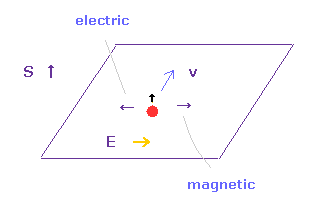In anomalous hall effect, magnetic fields of internal ferromagnetism play the same role as external magnetic field B in hall effect.
So they insist this effect is generated by spin-orbit interaction.
( Because they insist ferromagnetism is due to unrealistic "spin". )

In nonmagnetic materials, this current is separeted into two opposite directions depending on their magnetic moment's directions ( = spin hall effect ).
As shown in Fig.37 an Eq.38, these effects are the result of the interactions between orbital magnetic moment ( NOT spin ) and currents.

As you see in this page, these experiments did NOT prove the existence of unrealistic "spin" at all.
So "spin" is just an "imaginary" concept.

## Appendix.

### [ Spin-spin interactive energy is unrealistically weak ! ]

(Ap.1)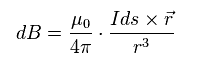According to Biot-Savart law, magnetic field (= B ) is as shown in Ap.1.
"I" is electric current, "ds" is electric current fragment, and "r" is the distance between this fragment and some point.

(Ap.2)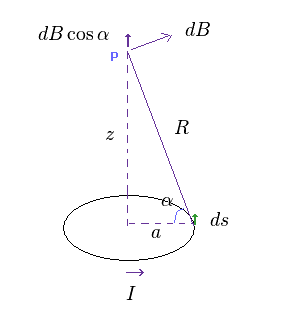We think about circular current of Ap.2.
The magnetic field B at the point "p" becomes the sum of the current fragments "Ids".
As shown in Ap.2, only cosine component of B is left after summing circular current.

(Ap.3)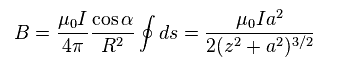where
(Ap.4)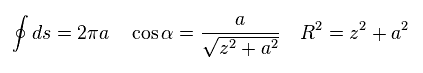Considering a single electron is very small, the radius "a" is much smaller than "z" in Ap.2.
So approximately, Ap.3 becomes,

(Ap.5)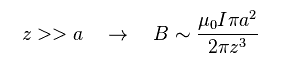Of course, even if you leave "a" in the denominator of Ap.3, this magnetic field is much smaller.
As shown in this page, Bohr magneton (= μB ) can be gotten by IS (= current × area ).

(Ap.6)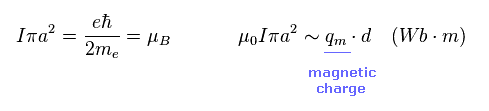As shown in Ap.6, magnetic moment is equal to magnetic charge (= q ) × distance (= d ).
This "d" is the short distance between "imaginary" ± magnetic charges "q" inside one magnetic moment.
( Of course, this is only an assumption. ± magnetic charges don't really exist. )

(Ap.7)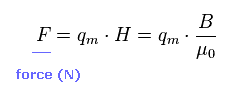The relation between force (N) and magnetic field is Ap.7.
In Ap.7, "B" is magnetic flux density ( Wb/m2 ), and μ0 is magnetic permeability.

(Ap.8)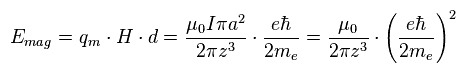When the distance between two spin magnetic moments is "z", the magnetic energy becomes as shown in Ap.8.
Because the energy is equal to magnetic force × distance "d" inside one spin .
(= Energy required to separate two ± magnetic charges q by "d" in magnetic field B. )

This magnetic force is given by Ap.7, and "B" is given by Ap.5.
( Of course, spin magnetic moment is Bohr magneton of Ap.6. )

(Ap.9)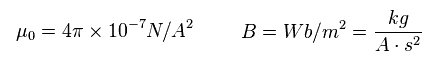SI units of permeability and magnetic flux density are as shown in Ap.9.

(Ap.10)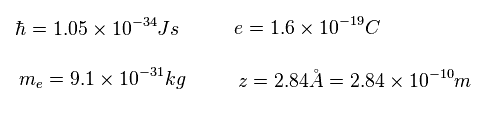Using Ap.9 and Ap.10, we compute Ap.8.
The result is

(Ap.11)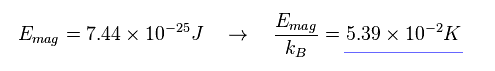Dividing the energy by Boltzmann constant kB, we find that this spin-spin interaction is extremely weak ( 0.0539 kelvin ).
If ferromagnetism is caused by spin-spin interaction, this magnet is easily broken in room temperature.
So this result shows "ferromagnetism" is caused by completely other things different from "spin".2013/9/10 updated. Feel free to link to this site.